Courses

# Retro (Past 13 Years) IIT - JEE Advanced (States Of Matter)

## 12 Questions MCQ Test Chemistry for JEE Advanced | Retro (Past 13 Years) IIT - JEE Advanced (States Of Matter)

Description
This mock test of Retro (Past 13 Years) IIT - JEE Advanced (States Of Matter) for JEE helps you for every JEE entrance exam. This contains 12 Multiple Choice Questions for JEE Retro (Past 13 Years) IIT - JEE Advanced (States Of Matter) (mcq) to study with solutions a complete question bank. The solved questions answers in this Retro (Past 13 Years) IIT - JEE Advanced (States Of Matter) quiz give you a good mix of easy questions and tough questions. JEE students definitely take this Retro (Past 13 Years) IIT - JEE Advanced (States Of Matter) exercise for a better result in the exam. You can find other Retro (Past 13 Years) IIT - JEE Advanced (States Of Matter) extra questions, long questions & short questions for JEE on EduRev as well by searching above.
QUESTION: 1

Solution:
QUESTION: 2

### X and Y are two volatile liquids with molar weights of 10 g mol-1 and 40 g mol-1 respectively. Two cotton plugs, one soaked in X and the other soaked in V, are simultaneously placed at the ends of a tube of length L = 24cm, as shown in the figure. The tube is filled with an inert gas at 1 atm pressure and a temperature of 300 K. Vapours of X and Y react to form a product which is first observed at a distance d cm from the plug soaked in X. Take X and V to have equal molecular diameters and assume ideal behaviour for the inert gas and the two vapours.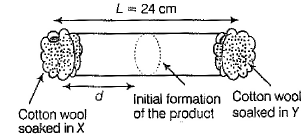Q. The experimental value of d is found to be smaller than the estimate obtained using Graham's law. This due to

Solution:
*Answer can only contain numeric values
QUESTION: 3

### If the value of Avogadro number is 6.023 x 1023 mol-1 and the value of Boltzmann constant is 1.380 x 10-23 JK-1, then the number of significant digits in the calculated value of the universal gas constant is (2014, Integer Type)

Solution:
QUESTION: 4

For one mole of a van der Waals' gas when b = 0 and T = 300 K, the pV vs 1/V plot is shown below. The value of the van der Waals' constant a (atm L mol-2)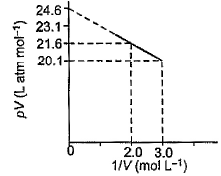Solution:
QUESTION: 5

According to kinetic theory of gases

(2011, Single Option Correct)

Solution:
According to a postulate of kinetic theory of gases, collision between the  molecules as well as with the wall of container is perfectly elastic in nature.

Hence, option (a) is correct

*Answer can only contain numeric values
QUESTION: 6

To an evacuated vessel with movable piston under external pressure of 1 atm, 0.1 mole of He and 1.0 mole of an unknown compound (vapour pressure 0.68 atm at 0°C) are introduced. Considering the ideal gas behaviour, the total volume (in litre of the gases Of 0°C is close to)

(2011, Integer Type)

Solution: Let nx mole of unknown compound be present in vapour form so its partial pressure can be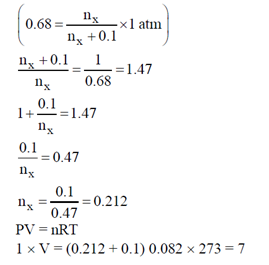QUESTION: 7

The term that corrects for the attractive forces present in a real gas in the van der Waals' equation is

(2009, Single Option Correct)

Solution:
*Answer can only contain numeric values
QUESTION: 8

At 400 K, the root main square (rms) speed of a gas X (molecular weight = 40) is equal to the most probable speed of gas y at 60 K. The molecular weight of the gas y is

(2009, Integer Type)

Solution:
QUESTION: 9

A gas described by van der Waals' equation

(2003, Single Option Correct)

Solution:
QUESTION: 10

Match the gases under specified conditions listed in Column I with their properties/laws in Column lI.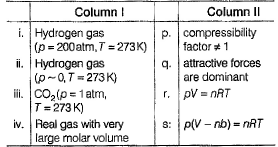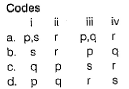Solution:
QUESTION: 11

The given graph represent the variations of Z (compressibility factor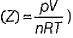versus p, for three real gases A, B and C.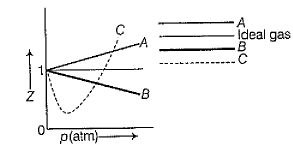Identify the only incorrect statement.

(2006, Single Option Correct)

Solution:
QUESTION: 12

If helium and methane are allowed to diffuse out of the container under the similar conditions of temperature and pressure, then the ratio of rate of diffusion of helium to methane is

(2005, Single Option Correct)

Solution: